Emblem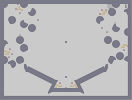Hover over the thumbnail for a full-size version.

Author Qix action author:qix playable unrated 2008-05-23 4 more votes required for a rating. \$Emblem#Qix#none#=00000000:I00:=00000000000;L000000000CH0000000000:=000000000:=000000000000000;<000;<00000000<000;<00:=;<0:E00000000=000>=0000:E000JL0000000G<00;<00000000>1@000000:=00:=000000000BD000000000000000000000>1@000000000000000000000BD000000000000000000000>1@000000000000000000000BDGKA000000000000000000>1M100000000000000000000I0100000000000000000000001000000000000000000000010000000000000000000000100000000000000000000H010000000000000000000?1L10000000000000000000CEFJ@00000000000000000?1A00000000000000000000CE00000000000000000000?1A0000000;<00000000000CE00000000:A0000000000?1A0000000000000;<00000KM0000000;<00000:=000K<00000000<:=00K<00;<00:I00000000=0000>=00:=0;<000000000000000000000:=0000000000CH000;<00000;@00000000|5^756,36!12^600,84!12^612,60!12^636,60!0^624,84!0^636,72!0^624,72!0^612,84!12^744,300!12^768,276!0^744,270!0^756,270!0^744,282!0^732,282!10^396,252!12^396,528!0^438,516!0^426,516!0^366,516!0^354,516!0^342,510!0^336,522!0^450,510!0^456,522!12^432,498!12^360,498!12^108,24!12^96,54!12^120,78!0^114,42!0^120,54!0^132,60!0^108,54!12^642,192!12^612,168!12^72,324!12^60,294!0^42,312!0^30,306!0^36,324!0^48,324!12^54,342!0^54,312!9^690,138,1,1,20,5,0,0,-1!9^120,210,1,1,12,5,0,0,-1!12^228,120!11^660,36,396,462# Based off of part of a symbol. Kind of tricky.

Other maps by this author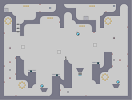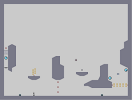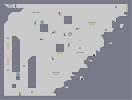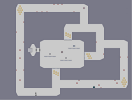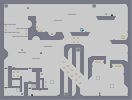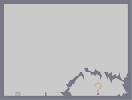A Fishy Recluse Succint Disintegrate Circuit Where are we going? 09-2:Lost Cause